Programming Geek
Rated 4.7/5 based on 1446 reviews### CodeVita 2016 Round 2 Question: Mate In Two

Background

A Chess board position is accurately captured by Forsyth-Edwards notation and is abbreviated as FEN. A FEN "record" defines a particular game position, all in one line of text and using only the ASCII character set. A FEN record consists of six fields. A complete description of the FEN format to represent Chess positions can be found here

For the purpose of this problem, only consider first of the six fields of FEN. Before we describe the problem, let us look at how FEN maps to a board position. The following 5 images show board positions and its corresponding FEN representation.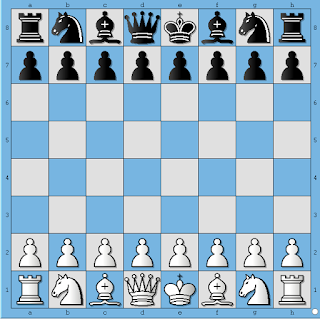Figure 1.

 This board position depicts initial position before any side has made a move. In FEN format this board position is represented as rnbqkbnr/pppppppp/8/8/8/8/PPPPPPPP/RNBQKBNR

Let's say, White plays e4. Then the board position looks like shown below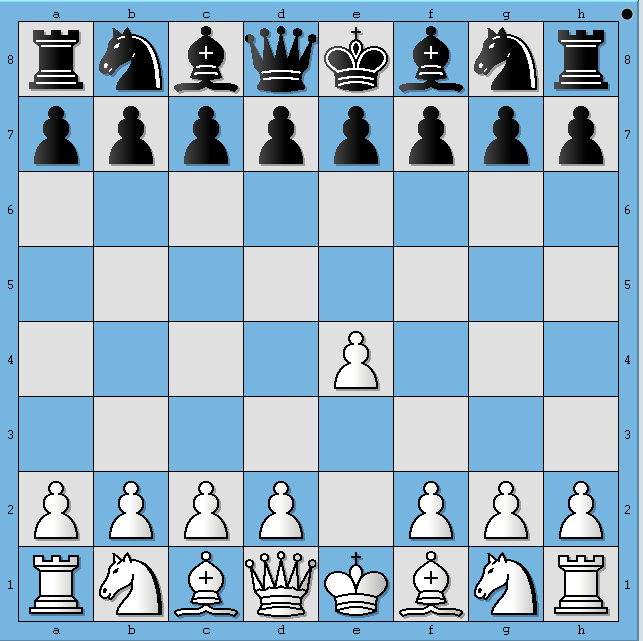Figure 2.

 This board position depicts the Chess board after White has played e4. In FEN format this board position is represented as rnbqkbnr/pppppppp/8/8/4P3/8/PPPP1PPP/RNBQKBNR

Similarly, 3 more half-moves are depicted in following diagrams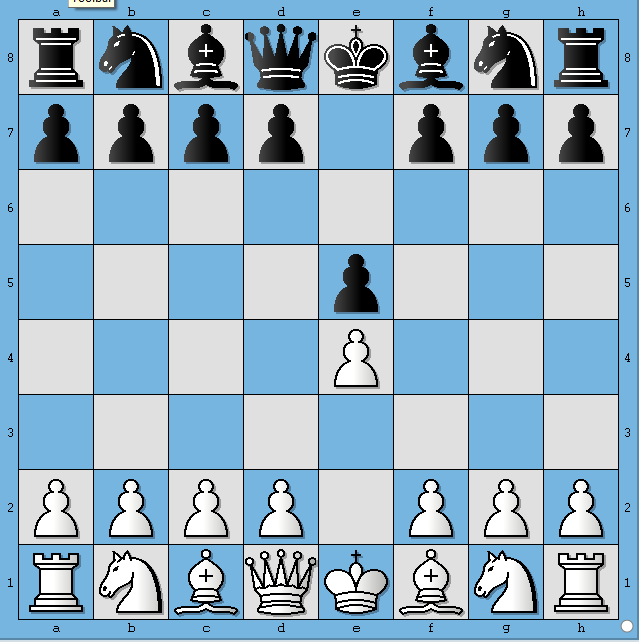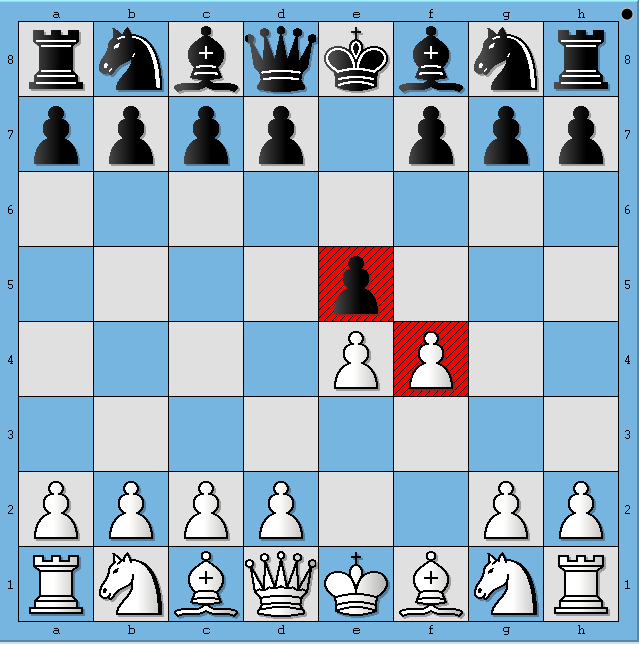Figure 3. Figure 4. Figure 5.

The FENs corresponding to Figure 3, 4 and 5 are represented as

3. rnbqkbnr/pppp1ppp/8/4p3/4P3/8/PPPP1PPP/RNBQKBNR
4. rnbqkbnr/pppp1ppp/8/4p3/4PP2/8/PPPP2PP/RNBQKBNR
5. rnbqkbnr/pppp1ppp/8/8/4Pp2/8/PPPP2PP/RNBQKBNR

Wikipedia describes first field of FEN format as follows

Piece placement (from white's perspective). Each rank is described, starting with rank 8 and ending with rank 1; within each rank, the contents of each square are described from file "a" through file "h". Following the Standard Algebraic Notation (SAN), each piece is identified by a single letter taken from the standard English names (pawn = "P", knight = "N", bishop = "B", rook = "R", queen = "Q" and king = "K"). White pieces are designated using upper-case letters ("PNBRQK") while black pieces use lowercase ("pnbrqk"). Empty squares are noted using digits 1 through 8 (the number of empty squares), and "/" separates ranks

Statement

Given a board position in FEN format, your task is to find out all move(s) that lead to a forced mate. White to play and win in 2 moves.

Input Format:
1. First line contains single FEN record, which corresponds to a particular board position

Output Format:
1. The output must be printed as follows
1. A string in format by "< move format >-< move format >-< move format >", where first move is white move, second is black move and third is again a white move
2. Where < move format > is move represented in format "fromSquaretoSquare"
2. See Example section for better understanding of output format

Constraints:
1. The board position will always be White to move and mate in 2
2. Since we focus on only first part of the FEN, we are essentially ignoring possibility of Castling being a mating move. Hence our test cases don't contain FENs which give rise to such positions.
3. There is no need to handle En Passant positions. There are no test cases involving En Passant moves.
4. No need to implement pawn promotion rules. Our test cases do not contain positions which will lead to a pawn getting promoted and inflicting a mate.
5. There is exactly one forced mating sequence in our test cases. So once a forced mating sequence is found, there is no need to process further.

Sample Input and Output

SNo.InputOutput
1
r1b2rk1/1p4pR/p2pppn1/6q1/3NP1P1/2N2P2/PPP4Q/1K5R

h7g7-g8g7-h2h7
2
5r1k/p4p1p/5P1N/1p1p4/2pP3P/8/PP4RK/8

g2g8-f8g8-h6f7

Explanation:

 Board position for sample input 1: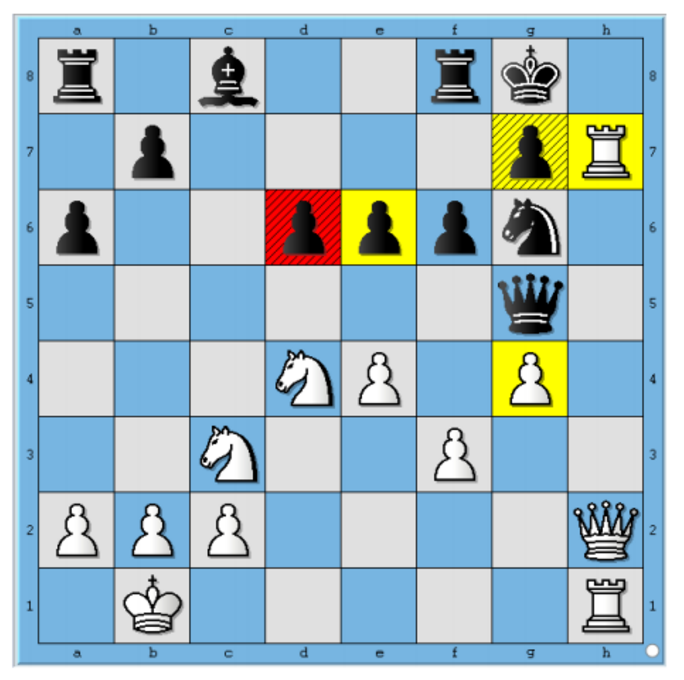Figure 6. Mate enforcing moves for input 1:Figure 7. Board position for sample input 2:Figure 8. Mate enforcing moves for input 2:Figure 9.### CodeVita 2016 Round 2 Question: Decrypt the Crypt

Problem : Decrypt the Crypt

Statement

Walter uses multiple applications at his workplace. He was assigned a unique password of variable lengths for each application (maximum length being 10). The only common factor among them was that all the passwords were a combination of 10 distinct characters. Walter created an algorithm to encrypt these passwords and wrote the codes on his desk to avoid a situation in which he would not be able to recollect a password.

Given as an input, is an encoded password and a list of 10 unique characters. Write a program that obtains the password by reverting the algorithm used by Walter.

Algorithm used by Walter to encode his password is as follows:
1. Walter observes all his passwords and lists down every distinct character. He assigns an index value to each character from 0 to 9. (Note: This list will be provided as an input to the program). This list MUST contain 10 DISTINCT characters.
2. He then replaces every character in the password with the corresponding index value to convert the password to a 10 digit numeric value.
3. The leftmost digit in this number sequence is noted; let's say 'A'. Now, starting from the leftmost position, every digit in the number is added to the digit on its right. The last digit in the sequence is added to 'A'.
4. Step 3 provides new sequences of numbers. He then scans through each of the summations and if any of the summation results in a value greater than 9, it is subtracted by 10, and its position is noted (Say B).
5. He then lists every digit from 0 to 9 separated by '|' character and prepends each digit with its position in the sequence as noted in 'B'. The resulting sequence after this step is saved (Say C).
6. The encoded password would therefore be
< value_of_C >||< contents_of_array_B  > < Value_of_A >

Decrypt the encrypted password that has been created by Walter using the above algorithm to compute the actual password!

Print -1 if there is any error in the inputs.

Input Format:
1. First line contains the encoded password as it should be according to Walter's algorithm
2. Second line contains 10 unique characters

Output Format:

Obtained password by reverting the algorithm. Print -1 if there is any error in the inputs.
Constraints:
1. Second line of input MUST contain 10 DISTINCT characters.
2. Encoded password should be in format
< value_of_C >|| < contents_of_array_B >

Sample Input and Output

SNo.InputOutput
1
0|1|2|43|14|5|6|7|308|29||0149
*Acf\$Zd&T@

@@Z\$\$
2
0|1|2|43|14|5|6|7|308|29||0149
*Acf\$Zd&T

-1

Explanation of Test Case 1:

NOTE: - The following are the steps used to encrypt the password. Your task is to reverse the logic and decrypt the encrypted string provided as input, and get back the clear-text password.
1. Consider input characters and its position

 Input character list : *Acf\$Zd&T@ Position : 0 1 2 3 4 5 6 7 8 9

(Note: The character list will not contain more than 10 characters and each character must be unique)
2. Now replace the characters in the password with the position value of the respective character as defined in step 1, to convert the password into a numeric value.

 Password : @@Z\$\$ Numeric value : 9 9 5 4 4

(Note: Remember leftmost value (Say A) = 9)
3. Starting from the left most position, add each digit to the digit on its right. Add the last digit with the first digit in the sequence

 : :
 9 9 5 4 4 18 14 9 8 13

4. For each addition result equal to or greater than 10, subtract it by 10 and note its position.

 Additive step output Selective subtraction Numeric value
 : : :
 18 14 9 8 13 8 4 9 8 3 0 1 4

(Note: Remember the selective subtraction step positions obtained (Say B) = 014)

 Format : 0|1|2|3|4|5|6|7|8|9
6. Now, each number in this format is pre-appended with the positions of the occurrence of the respective number in the output obtained in selective subtraction step of Step 4.

(Note: Say C = 0|1|2|43|14|5|6|7|038|29)
7. The final encrypted password will be of the following format to account for the calculations performed in generating the encrypted password

 Encrypted password (format) : < C >||< B >< A > Encrypted password : 0|1|2|43|14|5|6|7|308|29||0149

Explanation of Test Case 2:

Second line of input contains 9 characters only hence prints -1### CodeVita 2016 Round 2 Question: Crack the Password

Statement

Atul does lots of online transactions and has accounts in multiple banks, but to remember his passwords he created his own encryption technique and wrote down the enciphered passwords in a notepad. But he finds it very time consuming to decipher those passwords so he has asked for your help to develop a program to do the task quickly.

The approach he takes to encipher is,
1. First he writes down his password in a square matrix P of dimension N, sequentially from the first element (see example for better explanation). He chooses N such that N^2 is the least number above the length of the password. He then starts filling the matrix row-wise from top to bottom. If any matrix element remains blank, he fills all those blanks with the last element e.g. if the last element filled is z then he fills all other remaining elements in the matrix by z .
2. Then he creates new matrix A of the same dimensions such that every A(i,j) = P(j,i). Next he assigns the value of the alphabet in the password to that corresponding element in the matrix. So 'a' is substituted by 1, 'b' by 2, so on. Alphabets are only lower case. Also passwords contain characters from a to z only.
3. Then he finds another matrix B such that, the solution to the following equation gives a matrix with all the elements of principal diagonal as 1 and rest as 0, which is also called the eMat( encrypted matrix ) .

Your are provided with the eMat (encrypted matrix) or the matrix B, your task is to find all possible passwords and print them.

Example:
Let the password be "passwords" as it has 9 characters , the most appropriate matrix will be a 3X3 matrix, hence the matrix will be

After Step 1:

After step 2:

After step 3:

The eMat for the given matrix will be

 -0.783784 0.189189 0.702703 -0.118503 0.0790021 0.0956341 0.877339 -0.251559 -0.725572

Input Format:
1. First line contains integer N, which is the dimension of square matrix eMat
2. Next N lines contain N space separated values (11-digit precision after decimal point) representing the eMat.

Output Format:

In a single line print the password, if multiple password values are possible print them separated by space, sorted by ascending order of their lengths.
Constraints:

1. When eMat is converted to Matrix A, round off the numbers in the matrix A to its nearest integer value to recover the alphabets in the password

Sample Input and Output

SNo.InputOutput

1

3
-0.78378378378 0.18918918919 0.70270270270
-0.11850311850 0.07900207900 0.09563409563
0.87733887734 -0.25155925156 -0.72557172557

2

2
0.06666666667 -0.06666666667
-0.00350877193 0.05614035088

pas pass### CodeVita 2016 Round 2 Question: Verify JSON Object validity

Problem : Verify JSON Object validity

Statement

A JSON object is a key-value pair data structure that is enclosed within { }. A sample JSON object would look like
{
"key1":"value1",
"key2":"value2",
"key3": {
"key4":"value4",
"key5":"value5"}
"key6":"value6",
"key7":[
{
"key8":"value8"
}]
}

Given a JSON object, ignore the literal values of the object and check whether the distinguishing characters and notation of the object are valid to determine if the JSON object is valid or not.

Note:
1. Key3 points to another JSON object (Concept of nesting of JSON objects).
2. Key7 points to an array of JSON objects.
You may wish to refer site1 to get a more formal description of JSON grammar. site2,site3; are also good resources to understand JSON specifications.

Input Format:
1. First line contains a pattern of JSON without any literal

Output Format:

Print 1 if pattern is valid, -1 otherwise.
Constraints:
1. A JSON object should start with '{' and ends with a '}'.
2. The key and value should be separated by a ':'.
3. A ',' suggests an additional JSON property.
4. An array only consists of JSON objects. It cannot contain a "key":"value" pair by itself.

Example 1:

Input
{:[{},{}]}

Output
1

Explanation
{
"Key": [{
"Key": "Value"
}, {
"Key": "Value"
}]
}
Pattern is following all constraints hence prints 1
Example 2:

Input
{:{[]},{}}

Output
-1

Explanation
Convert this pattern in a JSON Object

{
"Key": {
[
"Key": "Value"
]
},
{
"Key": "Value"
}
}

Constraint 4 "An array only consists of JSON objects. It cannot contain a "key":"value" pair by itself." not followed here, so it's a invalid pattern, hence prints -1### CodeVita 2016 Round 2 Question: The Mystery of Sky

Problem : The Mystery of Sky

Statement

Stark is a 10 year old kid and he loves stars. So, he decided every day he will capture a picture of a sky. After doing this for many days he found very interesting observations.

Every day the total number of stars in the sky is same as days completed for a calendar year. He noticed, on Saturday's and Sunday's that there are no stars in the sky. Stark's camera does not have wide angle capture feature so he could only capture maximum of 50 stars at a time. So, he assumed that there are only 50 stars in the sky that day. Also, the camera discharges every 4th day and he is not be able to click any picture that day. So let's say, if the first day of calendar (01/01/0001) starts on a Monday then on Thursday he can't click any pictures. Then resuming on Friday he can take pictures until Sunday, but can't take picture on Monday, followed by downtime on Friday, then Tuesday, then Saturday etc. When the camera discharges he considers 0 stars that day.

You are his programmer friend and want to help him. You need to write a code which will tell him on a particular date how many stars Stark's camera was able to click.

You can assume Stark has an ancient camera and your first input will be the day for date (01/01/0001) and then followed by any date on which Stark wants to find out the number of stars in the sky.
Input Format:

Every line of input will contain a Day at date 01/01/0001 in dd/mm/yyyy format followed by a Date in the same format (on which we have to count the stars)
Output Format:
For valid Input
Count of the number of stars in the sky on the given date

For Invalid Input
Print "Invalid Date" for invalid date

Print "Invalid Day" for invalid day

Sample Input and Output

SNo.InputOutputExplanation
1
Monday
30/02/1990

Invalid Date

2
Thursday

Invalid Day

3
Wednesday
24/01/2056

24

Its 24th day of the year and neither is Saturday/Sunday nor has the camera discharged on this day.### CodeVita 2016 Round 2 Question: Collecting Candies

Problem : Collecting Candies

Statement

Krishna loves candies a lot, so whenever he gets them, he stores them so that he can eat them later whenever he wants to.

He has recently received N boxes of candies each containing Ci candies where Ci represents the total number of candies in the ith box. Krishna wants to store them in a single box. The only constraint is that he can choose any two boxes and store their joint contents in an empty box only. Assume that there are infinite number of empty boxes available.

At a time he can pick up any two boxes for transferring and if both the boxes say contain X and Y number of candies respectively, then it takes him exactly X+Y seconds of time. As he is to eager to collect all of them he has approached you to tell him the minimum time in which all the candies can be collected.
Input Format:
1. First line of input is number of test case T
2. Each test case is comprised of two inputs
1. First input of a test case is the number of boxes N
2. Second input is N integers delimited by whitespace denoting number of candies in each box

Output Format:

Print minimum time required, in seconds, for each of the test case. Print each output on a new line.
Constraints:
1. 1 ≤T≤10
2. 1 ≤N≤ 10000
3. 1 ≤ [Candies in each box] ≤ 100009

Sample Input and Output

SNo.InputOutputExplanation

1

1
4
1 2 3 4

19

4 boxes, each containing 1, 2, 3 and 4 candies respectively.
Adding 1 + 2 in a new box takes 3 seconds
Adding 3 + 3 in a new box takes 6 seconds
Adding 4 + 6 in a new box takes 10 seconds
Hence total time taken is 19 seconds. There could be other combinations also, but overall time does not go below 19 seconds.

2

1
5
1 2 3 4 5

33

5 boxes, each containing 1, 2, 3, 4 and 5 candies respectively.
Adding 1 + 2 in a new box takes 3 seconds
Adding 3 + 3 in a new box takes 6 seconds
Adding 4 + 5 in a new box takes 9 seconds
Adding 6 + 9 in a new box takes 15 seconds
Hence total time taken is 33 seconds. There could be other combinations also, but overall time does not go below 33 seconds.### CodeVita 2016 Round 2 Question: The Vita Sum

Problem : The Vita Sum

Statement

Tom the cat is brushing up his Math skills. He has a bag containing N balls of different colors. Now Tom can randomly pick any even number of balls from the bag. Tom wants to find out the sum of all such combinations of balls that he can pull out from the bag. He can pull out at max K balls in one pick.
Input Format:

First line contains two space separated numbers N and K
Output Format:

The output is the sum of all the combinations of balls he can pull out modulo 10^9+7 i.e. (1000000007)
Constraints:
1. 0<=N,k<=10^14
2. N >= k

Sample Input and Output

SNo.InputOutputExplanation

1

4 4

8

We need 4C0 + 4C24C4= 1+6+1=8

2

8 3

29

We need 8C0 + 8C2= 1+28=29### CodeVita 2016 Round 2 Question: Game of Marbles

Problem : Games of Marbles

Statement

Darrell and Sally are two best friends. They had a large collection of marbles. They devised a game with it to play in their free time which will also help them to improve their math. One of them will have to select a certain number of marbles and give a hint to find the number. The other will have to guess the first number that matches the given criteria and vice versa.

Your task is to act as a judge for this game. When the player finds the answer, you will have to verify the answer. If answer is right, add 10 points to that player. If the player passes the question, you will have to give the right answer (no change in points in this case). You should also announce the winner at the end of the game.

Hint to find the number:

When the marbles are put into a group of x1, x2, x3,...(where x1, x2, x3 can be any number from 1 to 100), it falls into a perfect group.(No marble is left without a group).

Example: - When Darrell says the number falls into a perfect set when she groups them into sets of 3 and 5, the answer could be 15 or 30 and so on. Since the first number that matches the criteria is 15, 15 will be the answer.(Explanation: when 15 marbles is put into groups of 3, We will get 5 sets of 3 marbles each and when it is put into groups of 5, we will get 3 sets of 5 marbles each. For 16 marbles, we will get 5 sets of 3 marbles each and one marble will be left without a proper group. So 16 cannot be the answer)

NOTE: - Please have a look at Sample Input and Output before you read the Input and Output specification
Input Format:

The input will contain
1. Number of input lines N adhering to the following specification
1. Lines will be of two types either a Question Posing line or Answer Giving line
2. Question posing line has to appear before any answer giving line
3. Question Posing line starts with Player Name and Answer Giving line starts with 'A'
2. Second line will be a Question posing line whose format is where Perfect Sets are depicted in the format <X1
3. ,X2,...Xn> where 2<=n<=7 and 1<=Xn<=100
4. Third line must be an Answer Giving line which is the answer to the precedingQuestion Posing line. The format of the Answer Giving line is as follows :
1. answer above can only be an integer number OR it will be a constant string "PASS"
2. An integer number represents the value of the answer given by the Player
3. If the Player does not know the answer she will "PASS" the question
5. Fourth line onwards, if they exist, will be alternating series of Question Posing and Answer Giving lines in case of Valid inputs
6. In case of any Invalid Question Posing line, requisite output must be printed for all previous Valid Question posing line(s).
7. Processing should stop at Invalid input line after printing required message in output. See output specifications and sample test cases to understand points 5) and 6) better

8. Output Format:
1. First line of output must reiterate the question in the following format < Player Name >'s question is : X1,X2,X3...,Xn>

9. Second line should be an evaluation of the first Answer Giving line of the input. The evaluation message will either be { Correct Answer or Wrong Answer}
1. is correct, output, : 10points
2. is wrong, output, : 0points
3. is "PASS"-ed by the player,
1. output "Question is PASSed"
2. output "Answer is: " where correct_answer_value is the correct answer for the question passed by the player.
3. output ": 0points"
4. Overall points collected by players have to be tracked and output when all valid inputs are processed
11. If all inputs are valid, after processing all the inputs, the final output should be comprised of the following 4 lines
1. Output "Total Points:" on fourth last line
2. Output ": points" on 3rd last line, where Player1 is the one who first posed the question
3. Output ": points" on 2nd last line, where Player2 is the one who first answered the question
4. If there is a winner Output "Game Result: is winner" or "Game Result: Draw"
12. Print "Invalid Input" in case of invalid input or failing constraint

13. Constraints:
1. 0 < N <= 10
2. Player Names are Case-sensitive
3. Number of inputs in a Question posing line will be 2<=n<=7 and 1<=Xn<=100
4. <X1 ,X2,X3...,Xn> can only be integers

Sample Input and Output

SNo.InputOutput

1

4
Sally 3,5
A Darrell 15
Darrell 4,8
A Sally 8

Sally's question is: 3,5
Darrell: 10points
Darrell's question is: 4,8
Sally: 10points
Total Points:
Sally: 10points
Darrell: 10points
Game Result: Draw

2

4
Darrell 5,6
A Sally 30
Sally 3,5
A Darrell PASS

Darrell's question is: 5,6
Sally: 10points
Sally's question is: 3,5
Question is PASSed
Darrell: 0points
Total Points:
Darrell: 0points
Sally: 10points
Game Result: Sally is winner

3

2
Darrell
A Sally 15

Invalid Input

4

4
Sally 3,5
A Darrell 15
Darrell
A Sally 15

Sally's question is: 3,5
Darrell: 10points
Invalid Input

5

2
Sally 3,5
A Darrell 3

Sally's question is: 3,5
Darrell: 0points
Total Points:
Sally: 0points
Darrell: 0points
Game Result: Draw

6

2
Sally 3,5,15
A Darrell 15

Sally's question is: 3,5,15
Darrell: 10points
Total Points:
Sally: 0points
Darrell: 10points
Game Result: Darrell is winner### TCS CodeVita 2016 Round 1 result

Congratulations to all selected people!